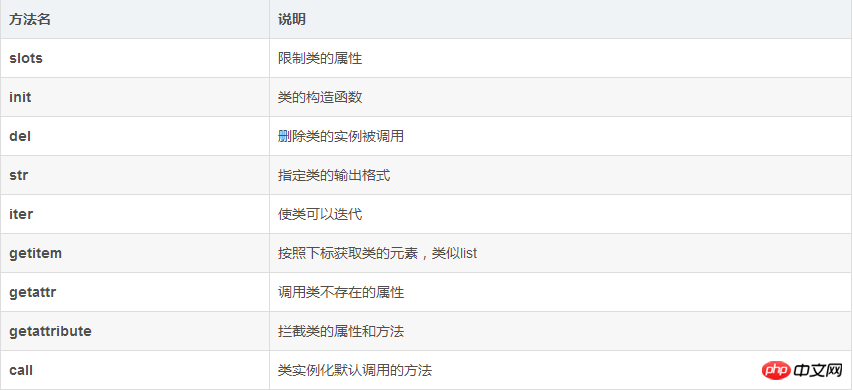# python定制类__str__（实例详解）

__slots__我们已经知道怎么用了，__len__()方法我们也知道是为了能让class作用于len()函数。__str__

```>>> class Student(object):
...     def __init__(self, name):
...         self.name = name
...
>>> print(Student('Michael'))
<__main__.Student object at 0x109afb190>```

```>>> class Student(object):
...     def __init__(self, name):
...         self.name = name
...     def __str__(self):
...         return 'Student object (name: %s)' % self.name
...
>>> print(Student('Michael'))
Student object (name: Michael)```

```>>> s = Student('Michael')
>>> s<__main__.Student object at 0x109afb310>```

```class Student(object):
def __init__(self, name):
self.name = name
def __str__(self):
return 'Student object (name=%s)' % self.name
__repr__ = __str__```